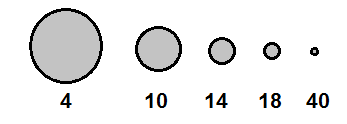﻿ Wire Gauges- Explained ﻿# Wire Gauges- ExplainedWire gauge is a measurement of a wire, either its diameter or cross-sectional area. The gauge of a wire determines how much current can flow through the wire. The gauge also determines the resistance of the wire and its weight per unit of length.

When dealing with wire gauge, the characteristics of a wire usually specified on a chart is the wire's size (specified in AWG), diameter, area, feet per pound, ohms per 1000ft, and current capacity (in amps).

Below is a chart of different wire gauges. All the terms associated with this table will be explained below:

 Wire Size (AWG) Diameter (MILs) Area (CM)2 Feet Per Pound Bare Ohms Per 1000ft 25°C Current Capacity (Amps) 4 204.3 41738.49 7.918 0.2485 59.626 8 128.5 16512.25 25.24 0.7925 18.696 10 101.9 10383.61 31.82 0.9987 14.834 12 80.8 6528.64 50.61 1.5880 9.327 14 64.1 4108.81 80.39 2.5240 5.870 18 40.3 1624.09 203.5 6.3860 2.320 20 32 1024.00 222.7 10.1280 1.463 22 25.3 640.09 516.3 16.2000 0.914 24 20.1 404.01 817.7 25.6700 0.577 28 12.6 158.76 2081 65.3100 0.227 32 8.0 64.00 5163 162.0000 0.091 40 3.1 9.61 34364 1079.0000 0.014

### Wire Size

The first column of the table is wire size. This is simply the size of the wire. In the table above, sizes 4-40 are included. Wire gauge of AWG 4 is very large in size. As we go down the chart, we decrease size more and more until we reach a wire size of AWG 40, which is a very small size. AWG stands for American Wire Gauge. The sizes 4-40 are standardized to this system and represent the diameter and area shown on the chart.The diagram above does not represent the exact size, but just a general diagram to show the approximate sizes of the type of gauges in reference to each other.

### Diameter

Diameter is the diameter of the wire. Since wire of AWG 4 is the largest of all the wires, it has the largest diamater. A wire size of AWG 40 has the smallest diameter, being it's the smallest wire.

Smaller wires always have greater resistance than larger ones. This is because there is less room for current to flow through. Current is simply the flow of electrons. When a wire is smaller, the electrons have less room to go through the wire. With less room, they bounce and rub off each other easier. This bouncing and rubbing produces friction, or resistance. In larger wires, there is more room. Therefore, electrons flowing bump and collide into each other less easier. Therefore, there is less friction, or resistance.

The diameter can be expressed in many ways, including in MILs, inches, or millimeters. 1 mil is equal to 0.001 in or 0.0254 mm.

### Area

The area of a wire is the area of its cross-section. This is the calculated by the typical area of a circle, A=πr2.

The area is specified in CM, circular MIL. A circular mil is a unit of area equal to that of a 1-mil-diameter circle. The CM area of a wire is the square of the mil diameter.

### Feet Per Pound

Feet per pound is the number of feet that a wire gauge will give you per 1lb of weight. For example, being that wire of AWG 4 is the largest wire, it is also the heaviest. Therefore, it takes only 7.918 feet of wire to weigh 1lb. Meanwhile, wire of AWG 40 is the smallest wire. Therefore, it takes 34,364 feet of wire to weigh 1lb. Feet per pound is a good measure to determine the weight of wire which will be used if the number of feet of the wire needed is known.

### Ohms Per 1000ft

Ohms per 1000ft is a measure of the resistance of a wire.

This can be used to determine the electrical resistance of a wire.

The longer the wire, the longer the distance. So with longer distance, there are more electrons bumping into each other as well as the walls of the wire. This creates friction and heat, which creates resistance. With greater resistance, this diminishes current capacity.

If the wire is shorter in length, this makes less resistance. If the wire is longer in length, this creates greater resistance.

In the table above, you can see that wire of AWG 4 has resistance of 0.2485Ω for 1000 feet, while wire of AWG 40 has a resistance of 1079Ω per 1000 feet. This is at 25°C.

### Current Capacity (Amps)

The current capacity of a wire is the amount of current which a wire can safely carry.

Larger wires, being able to accomodate more electrons, since it's larger, can carry much more current through them than smaller wires. So, you can see, based on the table, that a wire of AWG 4 can carry 59.626 amps of current safely. A wire of AWG 40 can only carry 0.014mA of current safely.

If the amount of current that a wire carries exceeds its current capacity, the wire may overload and melt and can cause a fire. Therefore, it is fire safety hazard to exceed this rating and is very dangerous.

In electronics, the standard size of wires used for breadboard are of AWG 22. Since electronics normally deal with low voltage and low current circuitry, current flowing through normally does not exceed 1 amperes. Normally the current is somewhere in the milliampere range. If using higher current than this, use a wire of a AWG that has a current capacity greater than the current which will be used.

HTML Comment Box is loading comments...

﻿